# NCERT Solutions for Class 8 Maths Chapter 4 Exercise 4.1 – Practical Geometry

NCERT Solutions for Class 8 Maths Chapter 4 Exercise 4.1 – Practical Geometry, has been designed by the NCERT to test the knowledge of the student on the topic – Constructing a Quadrilateral – When the lengths of four sides and a diagonal are given

### NCERT Solutions for Class 8 Maths Chapter 4 Exercise 4.1 – Practical Geometry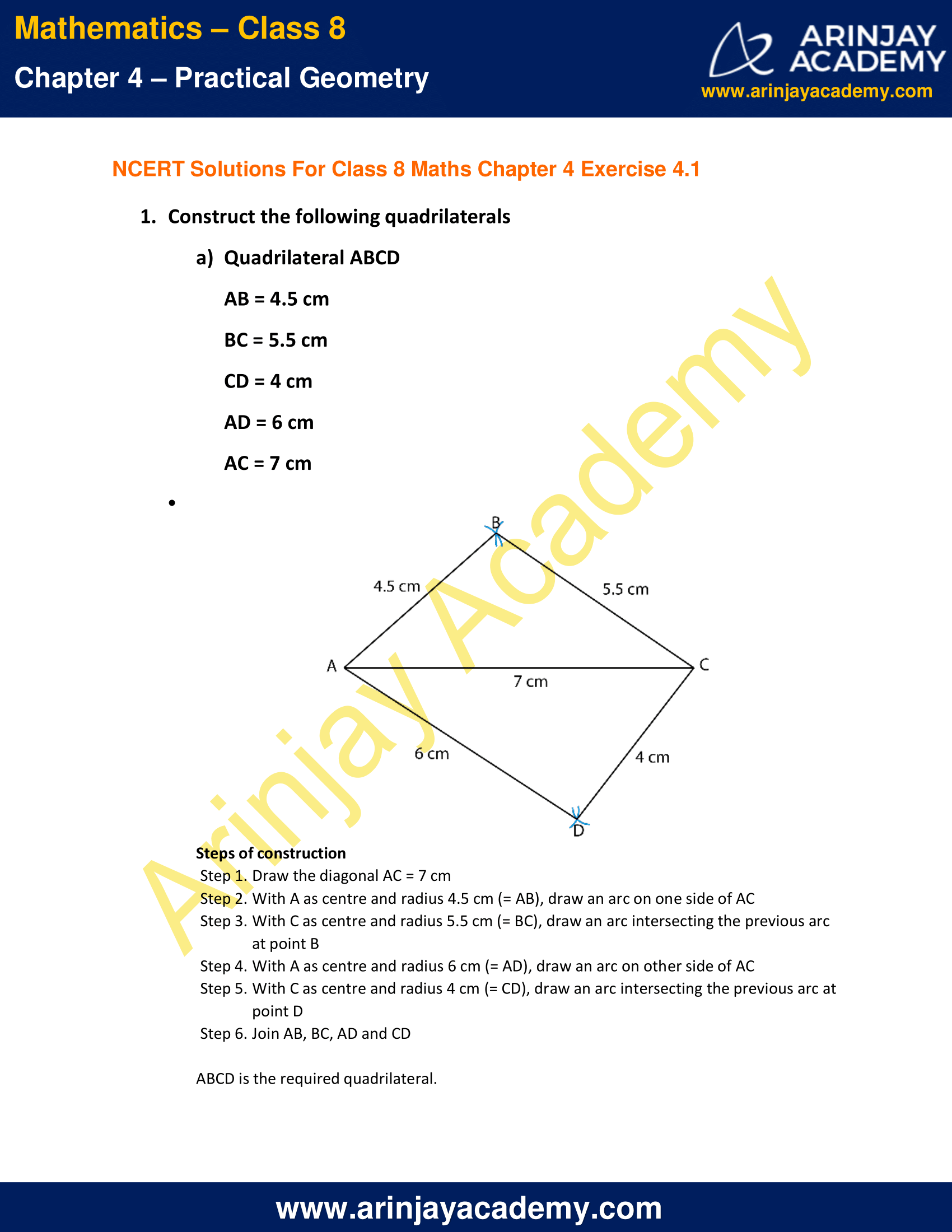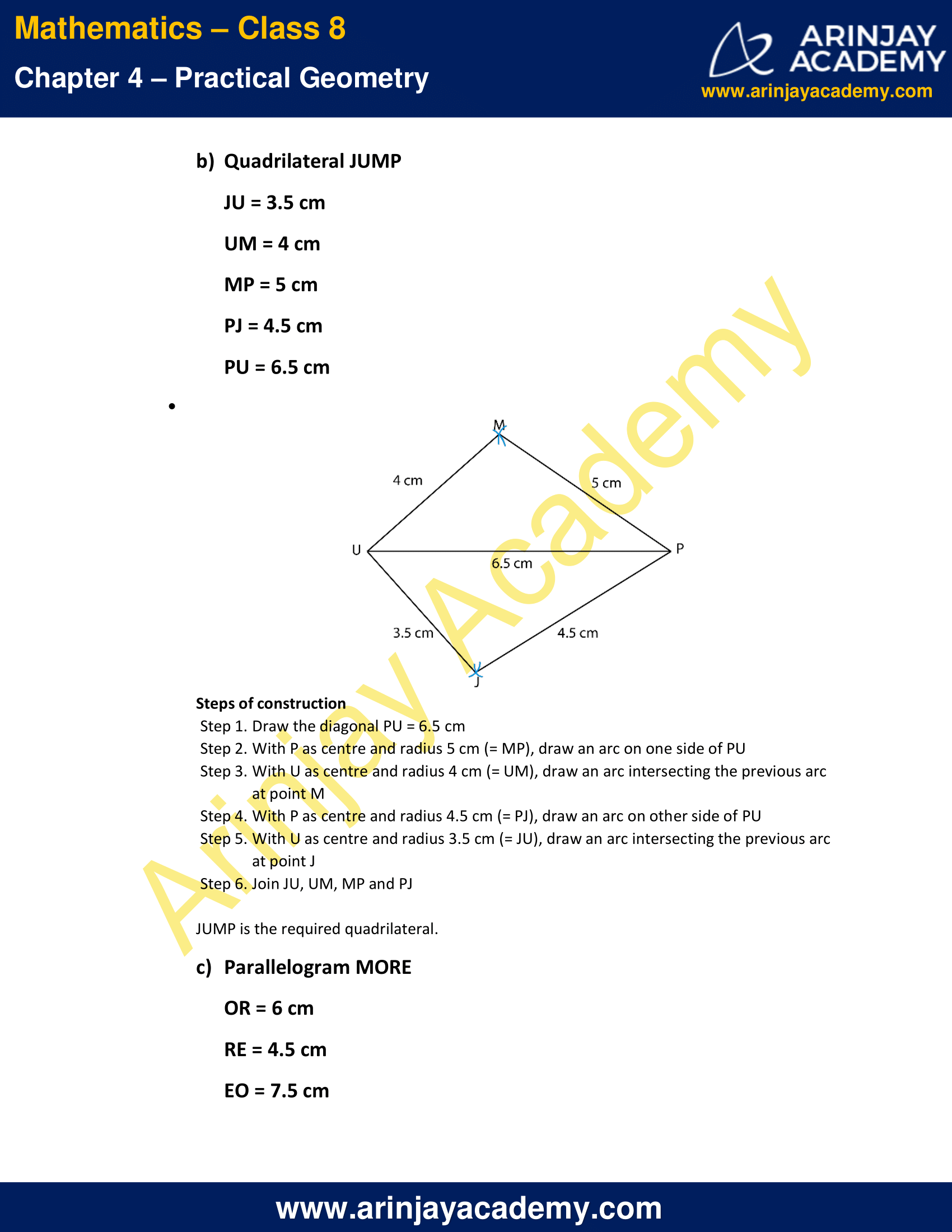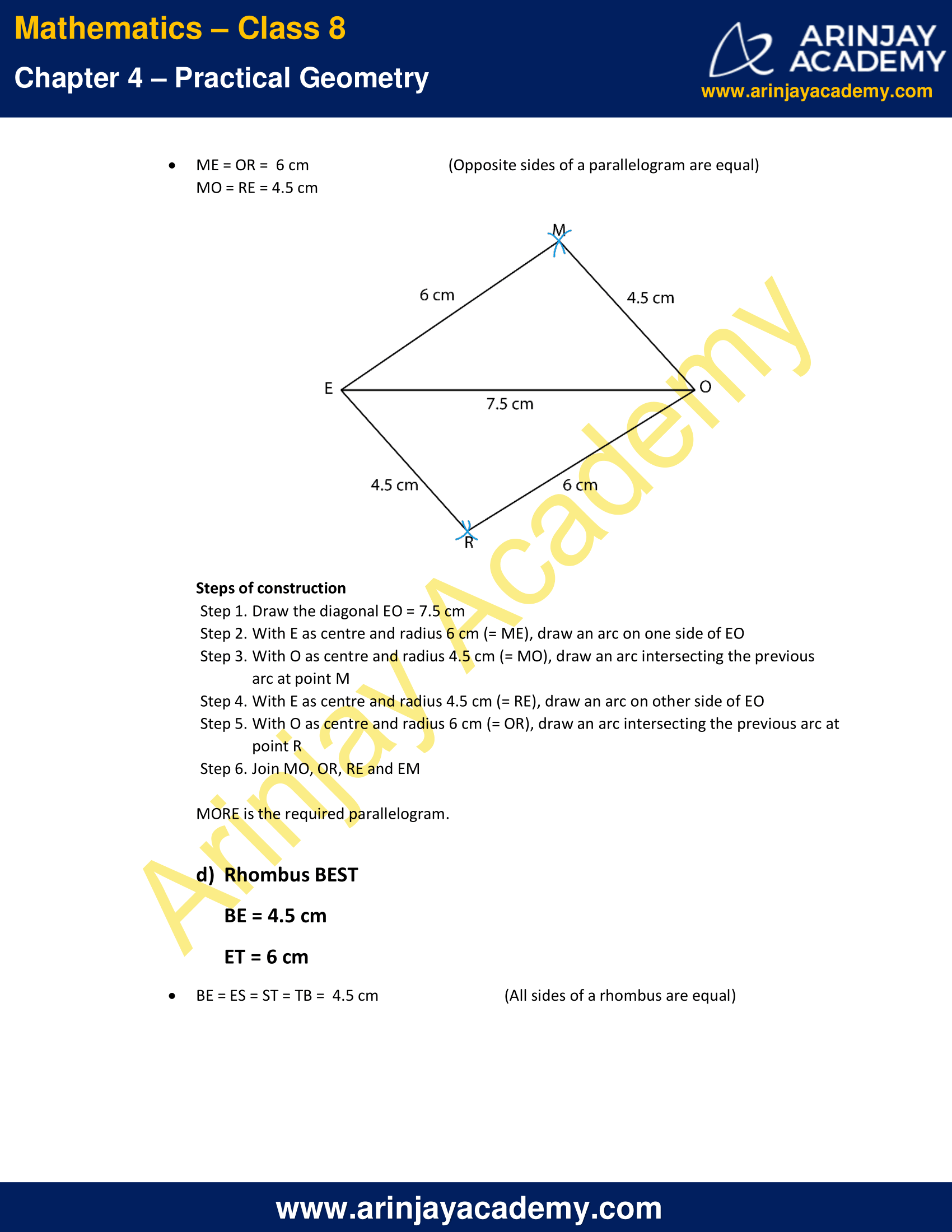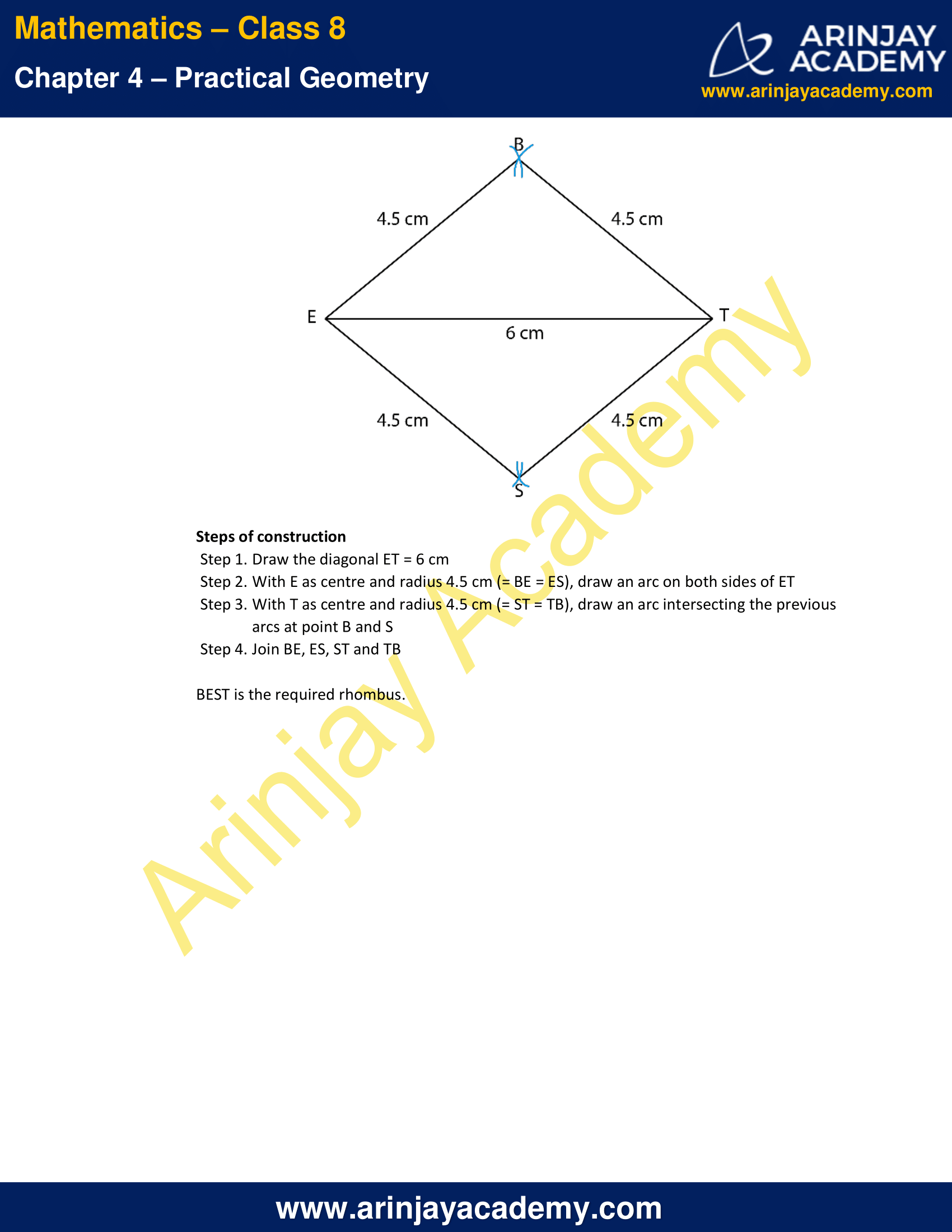NCERT Solutions for Class 8 Maths Chapter 4 Exercise 4.1 – Practical Geometry

AB = 4.5 cm
BC = 5.5 cm
CD = 4 cm
AC = 7 cm

Solution: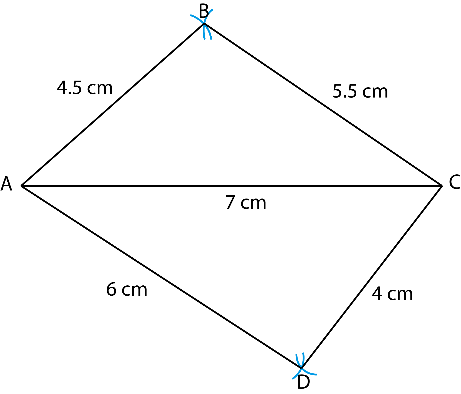Steps  for construction of a Quadrilateral

• Step 1. Draw the diagonal AC = 7 cm
• Step 2. With A as centre and radius 4.5 cm (= AB), draw an arc on one side of AC
• Step 3. With C as centre and radius 5.5 cm (= BC), draw an arc intersecting the previous arc at point B
• Step 4. With A as centre and radius 6 cm (= AD), draw an arc on other side of AC
• Step 5. With C as centre and radius 4 cm (= CD), draw an arc intersecting the previous arc at point D
• Step 6. Join AB, BC, AD and CD

JU = 3.5 cm
UM = 4 cm
MP = 5 cm
PJ = 4.5 cm
PU = 6.5 cm

Solution: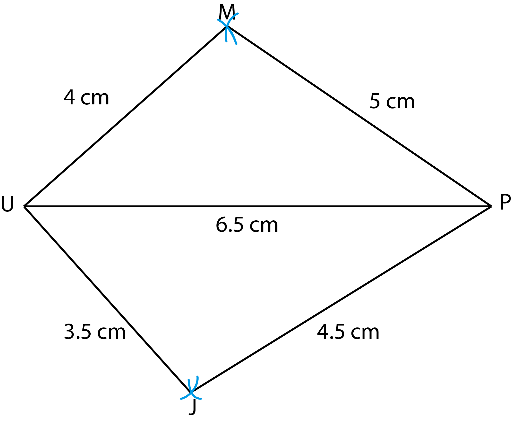Steps  for construction of a Quadrilateral

• Step 1. Draw the diagonal PU = 6.5 cm
• Step 2. With P as centre and radius 5 cm (= MP), draw an arc on one side of PU
• Step 3. With U as centre and radius 4 cm (= UM), draw an arc intersecting the previous arc at point M
• Step 4. With P as centre and radius 4.5 cm (= PJ), draw an arc on other side of PU
• Step 5. With U as centre and radius 3.5 cm (= JU), draw an arc intersecting the previous arc at point J
• Step 6. Join JU, UM, MP and PJ

iii. Parallelogram MORE

OR = 6 cm
RE = 4.5 cm
EO = 7.5 cm

Solution:

ME = OR = 6 cm  (Opposite sides of a parallelogram are equal)
MO = RE = 4.5 cmSteps  for construction of a Parellelogram

• Step 1. Draw the diagonal EO = 7.5 cm
• Step 2. With E as centre and radius 6 cm (= ME), draw an arc on one side of EO
• Step 3. With O as centre and radius 4.5 cm (= MO), draw an arc intersecting the previous arc at point M
• Step 4. With E as centre and radius 4.5 cm (= RE), draw an arc on other side of EO
• Step 5. With O as centre and radius 6 cm (= OR), draw an arc intersecting the previous arc at point R
• Step 6. Join MO, OR, RE and EM

MORE is the required parallelogram.

iv. Rhombus BEST

BE = 4.5 cm
ET = 6 cm

Solution:

BE = ES = ST = TB = 5 cm (All sides of a rhombus are equal)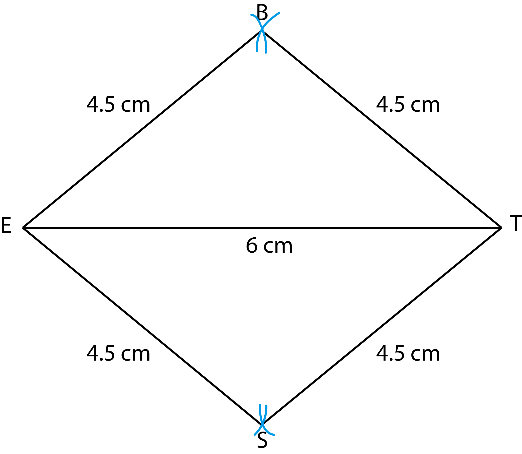Steps  for construction of a Rhombus

• Step 1. Draw the diagonal ET = 6 cm
• Step 2. With E as centre and radius 4.5 cm (= BE = ES), draw an arc on both sides of ET
• Step 3. With T as centre and radius 4.5 cm (= ST = TB), draw an arc intersecting the previous arcs at point B and S
• Step 4. Join BE, ES, ST and TB

BEST is the required rhombus.

The next Exercise for NCERT Solutions for Class 8 Maths Chapter 4 Exercise 4.2 – Practical Geometry can be accessed by clicking here

Download NCERT Solutions for Class 8 Maths Chapter 4 Exercise 4.1 – Practical Geometry